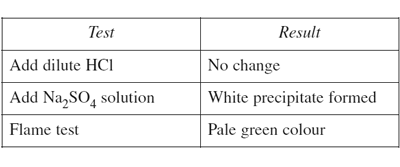General exercises (2010 NSW HSC) 1) Equal volumes of four 0.1 mol L−1 acids were titrated with the same sodium hydroxide solution. Which one requires the greatest volume of base to change the colour of the indicator? (A) Citric acid (B) Acetic acid (C) Sulfuric acid (D) Hydrochloric acid Solution 2) A sample of water from a stream, suspected to be contaminated with metal ions, was analysed. The results of some tests on the water are recorded in the table.What is the most likely contaminant in the water? (A) Ba2+ (B) Ca2+ (C) Cu2+ (D) Fe3+ Solution3) What mass of ethanol is obtained when 5.68 g of carbon dioxide is produced during fermentation, at 25°C and 100 kPa? (A) 2.95 g (B) 5.95 g (C) 33.6 g (D) 147.2 g Solution 4) Sodium azide is used in automobile airbags to provide a source of nitrogen gas for rapid inflation in an accident.The equation shows the production of nitrogen gas from sodium azide. 2NaN3(s) → 2Na(s) + 3N2(g) What mass of sodium azide will produce 40 L of N2 at 100 kPa and 0°C? (A) 70 g (B) 76 g (C) 114 g (D) 172 g Solution 5) Solutions containing copper ions were analysed by AAS. A standard solution of 10 ppm copper had an AAS absorbance of 0.400. A second solution of unknown concentration was found to have an absorbance of 0.500. 100 mL of this second solution was reacted with excess sodium carbonate solution. The precipitate was then dried and weighed. What mass of precipitate was formed? (A) 1.25 × 10−3 g (B) 2.43 × 10−3 g (C) 1.54 g (D) 2.43 g Solution6) A gas is produced when 10.0 g of zinc is placed in 0.50 L of 0.20 mol L−1 nitric acid. Calculate the volume of gas produced at 25°C and 100 kPa. Include a balanced chemical equation in your answer. Solution